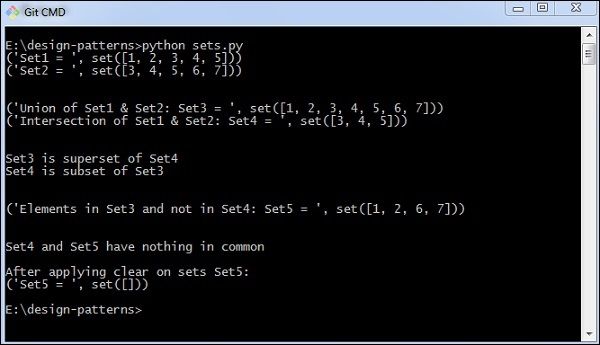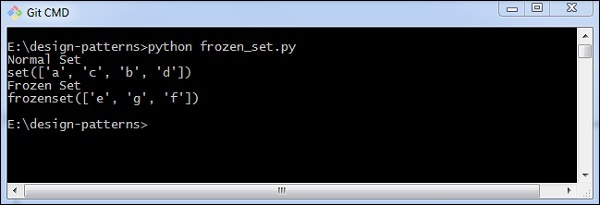# Python Design Patterns - Sets

A set can be defined as unordered collection that is iterable, mutable and there is no inclusion of duplicate elements in it. In Python, set class is a notation of mathematical set. The main advantage of using a set is that it includes highly optimized method for checking specific element.

Python includes a separate category called frozen sets. These sets are immutable objects that only support methods and operators that produce a required result.

## How to implement sets?

The following program helps in the implementation of sets −

```# Set in Python

# Creating two sets
set1 = set()
set2 = set()

for i in range(1, 6):

for i in range(3, 8):

print("Set1 = ", set1)
print("Set2 = ", set2)
print("\n")

# Union of set1 and set2
set3 = set1 | set2# set1.union(set2)
print("Union of Set1 & Set2: Set3 = ", set3)

# Intersection of set1 and set2
set4 = set1 & set2# set1.intersection(set2)
print("Intersection of Set1 & Set2: Set4 = ", set4)
print("\n")

# Checking relation between set3 and set4
if set3 > set4: # set3.issuperset(set4)
print("Set3 is superset of Set4")
elif set3 < set4: # set3.issubset(set4)
print("Set3 is subset of Set4")
else : # set3 == set4
print("Set3 is same as Set4")

# displaying relation between set4 and set3
if set4 < set3: # set4.issubset(set3)
print("Set4 is subset of Set3")
print("\n")

# difference between set3 and set4
set5 = set3 - set4
print("Elements in Set3 and not in Set4: Set5 = ", set5)
print("\n")

# checkv if set4 and set5 are disjoint sets
if set4.isdisjoint(set5):
print("Set4 and Set5 have nothing in common\n")

# Removing all the values of set5
set5.clear()

print("After applying clear on sets Set5: ")
print("Set5 = ", set5)
```

### Output

The above program generates the following output −The frozen set can be demonstrated using the following program −

```normal_set = set(["a", "b","c"])

# Adding an element to normal set is fine

print("Normal Set")
print(normal_set)

# A frozen set
frozen_set = frozenset(["e", "f", "g"])

print("Frozen Set")
print(frozen_set)
```

### Output

The above program generates the following output −